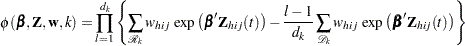Partial Likelihood Function for the Cox Model

Letdenote the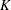distinct, ordered event times. Let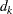denote the multiplicity of failures at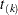; that is,is the size of the setof individuals that fail at. Let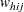be the weight associated with theth observation unit in theth cluster in stratum. Using this notation, the pseudo-likelihood functions used in PROC SURVEYPHREG to estimate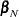are described in the following sections.

### Continuous Time Scale

Let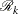denote the risk set just before theth ordered event time.

The Breslow likelihood is expressed as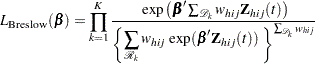The Efron likelihood is expressed aswhereis